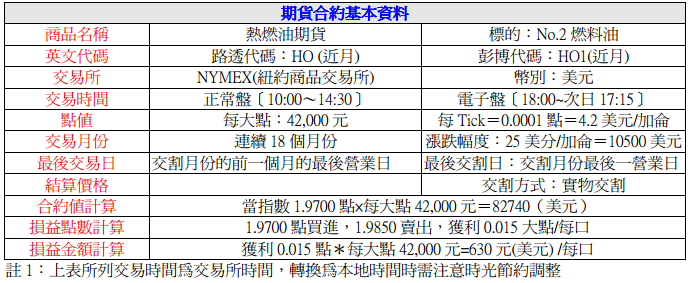## 2014年7月22日 星期二

### 外期 - 能源商品 - 熱燃油 [當沖與波段策略 ]

1978年熱燃油期貨合約推出為能源期貨市場開啟另一道里程碑。在石油分餾過程中，分餾油大約是在350~800度華氏蒸餾形成，為中間的提煉產品。分餾油可分為兩種：一號與二號油，一號油包括煤油和航空燃油，主要由石油的裂化殘渣油和直餾殘渣油製成的，其特點是粘度大，含非烴化合物、膠質、瀝青質多,通常用於爐具或燈具的燃料，而航空用油的沸點與一號油相近，一號油佔分餾油總量的15%左右，其餘為二號油，二號油又稱熱燃油，在歐洲地區則稱為製氣油(Gas Oil) ，柴油與熱燃油的性質相近，柴油可作為熱燃油使用，但熱燃油則不能作為柴油使用。二號油通常用於高緯度國家中的家庭或小型商業公司加熱取暖之用。

(一)原油價格走勢

(二)匯率影響

(三)利率的影響

(四) 經濟發展

(五)石油輸出國家組織（OPEC）生產動向

(六)地緣政治影響

(七)美國石油供需資料，特別是每週二美國石油協會(API)及周三美國能源部(EIA)公佈的每週能源供需狀況變動表。

(八)季節性因素

(九)庫存量高低

(十)煉解價差交易

(十一)替代品因素

(十二)市場參與者行為預測，如各國基金，油品公司，航空公司及一般投機客等。由於他們對基本面的理解更?深刻並具有"先知先覺"效果,其中基金更是造成近年油價大幅波動原因之一，因此瞭解基金的動向也是把握行情的關鍵。{ ***** System Code Start here *****}
{ Public inputs }
Inputs:Time2SW(0),Time2B(900),Time2E(1300),Time3SW(0) ;
Inputs:Time3B(900),Time3E(1300),PositionSW(0) ;
inputs:Frac_LMM(3.86),NBar_LM(16),Frac_SMM(1.06),NBar_SM(10) ;

{ Public Variables }
Vars:Cond_LE(false),Cond_SE(false),CondNet_L(false),CondNet_S(false),
Vars:TimeOK1(true),TimeOK2(true),TimeOK3(true),PosSW(true) ;

{ SellMode Setup }
inputs:Bar_S1(34) ;

{ Exit Long Position Setup }
{ Exit Short Position Setup }
{ SellMode Variable Setup }

{ Exit Long Position Variable Setup }
Vars:TL_ATR(0),Trigger_TLA(false) ;
Vars:LMM_ATR(0) ;

{ Exit Short Position Variable Setup }
Vars:TS_ATR(0),Trigger_TSA(false) ;
Vars:SMM_ATR(0) ;

{ initial profit and loss }
if MarketPosition = 0 then begin
end ;

{ ATR calculate for code }
LMM_ATR = AvgTrueRange(NBar_LM);
SMM_ATR = AvgTrueRange(NBar_SM);

{ Entry and Exit prices }
USell = Lowest(Open,Bar_S1) ;

{ Long and Short Entry Condition Setup }
Cond_LE = MOD(DayofMonth(Date),5) <> 1 ;
Cond_SE = (MOD(DayofMonth(Date),7) <> 1 or DayofMonth(Date) = 21) ;

{ Combine Trade Number in day }
Cond_LE = Cond_LE and EntriesToday(date) <= TradeInDay ;
Cond_SE = Cond_SE and EntriesToday(date) <= TradeInDay ;

{ Combine Trade time zone in day }
if Time1SW = 0 then TimeOK1 = true else TimeOK1 = (time >= Time1B and time <= Time1E) ;
if Time2SW = 0 then TimeOK2 = true else TimeOK2 = (time >= Time2B and time <= Time2E) ;
if Time3SW = 0 then TimeOK3 = true else TimeOK3 = (time >= Time3B and time <= Time3E) ;

Cond_LE = Cond_LE and TimeOK1 ;
Cond_SE = Cond_SE and TimeOK1 ;

{ Check Position status for entry}
if PositionSW = 0 then PosSW = true else PosSW = (MarketPosition = 0) ;
Cond_LE = Cond_LE and PosSW ;
Cond_SE = Cond_SE and PosSW ;

{ Entry Long orders }

{ Entry Short orders }
if Cond_SE then SellShort next bar at USell Stop ;

{ Exit orders, long trades }
If MarketPosition > 0 then begin
If BarsSinceEntry = 0 then begin
BuyStop = EntryPrice - Frac_LMM * LMM_ATR;
end;
Sell next bar at BuyStop stop;
end;

{ Exit orders, short trades }
If MarketPosition < 0 then begin
If BarsSinceEntry = 0 then begin
SellStop = EntryPrice + Frac_SMM * SMM_ATR;
end;
BuytoCover next bar at SellStop stop;
end;

if IntraDay = 0 then SetExitonClose

{***************** End of Strategy ************}